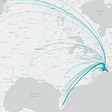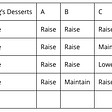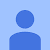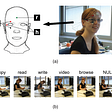# Part 1: Introduction to Dash

Every Dash app requires a layout. The layout includes:

• dash core components `dcc.Graph`
• dash html components `html.Div`, `html.H3`
`app.layout = html.Div(    children=[        html.Div(            className="data-class",            children=[                html.H3("head"),                dcc.Graph(id="my-id"),            ],        ),    ])`
• `html.Div` is more like a wrapper, and it can contain other components like `dcc.Graph` etc.

# Part 2: Notes

(1) if the ouput is `html.Div`, then its property is `children`

`html.Div(id="my-div")@app.callback(Output("my-div", "children"))def fun()  return html.Div([dcc.Graph(figure=fig)]) `

(2) if the output is `dcc.Graph`, then its property is `figure`

`dcc.Graph(id="my-graph")@app.callbackdef fun()  fig={     "data": [go.Bar()],     "layout":{ }  }or @app.callbackdef fun() from plotly.subplots import make_subplots fig = make_subplots(rows=2, cols=1, shared_xaxes=True,   ) fig.add_trace(go.Scatter(x=x_ax, y=a),              row=1, col=1) fig.add_trace(go.Scatter(x=x_ax, y=b),              row=2, col=1) fig.update_layout(height=900, width=900,                  title_text="process") return fig `

The second way of support file downloading is to put the generated files in `/assets/` directory, then use the following code to access the file:

• step 1: generate file in the denoted directory
`file_to_be_dowload = ""if os.path.exists(file_to_be_dowload):   pass else:   # generate the file `

This step is important because the generated files are located in assets directory, if every time we change files kept there, the Dash will refresh.

• step 2: generate the file link as html content
`text =  html.A(link_name, href=href_cont, target="_blank")return html.Div([text])`

# 3. 1 Figure.Data

There are several ways of generating figures:

(1) `make_subplots`

`from plotly.subplots import make_subplotsfig = make_subplots()fig.add_trace()fig.add_trace(go.Scatter())fig.update_layout()`

(2) `fig dict`

`fig = {   'data': [ go.Bar(), ]   'layout':{       'title': title_name,    }}`

(3) `go.Figure`

`fig = go.Figure()fig.add_trace()`

(4) `figure_factory`

`import plotly.figure_factory as fffig = ff.create_table(df)return html.Div([dcc.Graph(figure=fig)])`

or an example Figure Factory Tables in Python

# 3.2 Figure.Layout

The properties in the figure that can be updated include:

• height=
• width=
• title_text=
• yaxis={‘visible’: False, ‘showticklabels’: False}
• xaxis=
• showlegend=False

# Reference

--

--

--

Love podcasts or audiobooks? Learn on the go with our new app.

## Web Scraping## Prediction of Late Payments Using Decision Tree-Based Algorithms## Medical Tourism Financial Model## How to find an English teacher. Part 2.## Where did New York City hide from the pandemic?## How to think about combinatorics like a data scientist## Death in the Data Science Age## Preparing DNS Data for Cyber Security-Focused Data Science## Backend with Python: My Experience Part 2## Quick Start Guide to Technical Analysis## How the Monkees Set Me Up for Disappointment## Using eye movement analysis for activity recognition